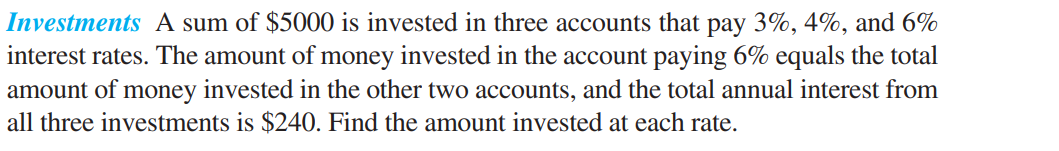### Still have math questions?Investments A sum of $$\ 5000$$ is invested in three accounts that pay $$3 \% , 4 \%$$ , and $$6 \%$$ interest rates. The amount of money invested in the account paying $$6 \%$$ equals the total amount of money invested in the other two accounts, and the total annual interest from all three investments is $$\ 240$$ . Find the amount invested at each rate.
$$\left. \begin{array} { l } { \text { from home: } \ 2687.50 \text { 11. } \ 1000 \text { at } 3 \% \text { ; } \ 1500 \text { at } 4 \% \text { ; } } \\ { \ 2500 \text { at } 6 \% \quad [ 5.3 ] \text { 12. } - 3 \quad \text { 13. } 59 } \end{array} \right.$$